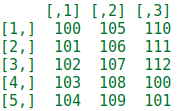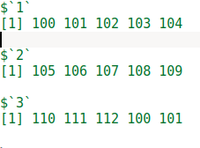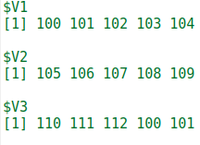Related Articles

# How to Convert matrix to a list of column vectors in R ?

• Last Updated : 21 Apr, 2021

In this article, we will discuss how to convert a given matrix to a list of column vectors in R Programming Language. To do this we will take every column of the matrix and store that column in a list and at last print the list.

It can be done in these ways:

• Using the split() function
• Using list() with data.frame()

Method 1: Using split() function:

In this example to Convert a given matrix to a list of column vectors in R we use the split() function.

• The split() function is used to split the data according to our requirement.
• The arguments given in the split() function are data itself and the other one is rep() function
• The rep() function is used to replicates the data and in the rep() function we give one argument i.e. nrow() so, that the sequence is generated row-wise
• In nrow() function we give the data as an argument. “each = nrow()” is used to repeat this process for each row.

Example:

## R

 `# create matrix``M = ``matrix``(100:112, ncol=3)`` ` `# print the matrix``display``(M)`` ` `# create matrix to list off column vector``l =  ``split``(M, ``rep``(1:``ncol``(M), each = ``nrow``(M)))`` ` `# print the list``display``(l)`

Output:fig 1: Matrixfig 2: Matrix converted to list of column vectors

Method 2: using the list() function:

In this example to Convert a given matrix to a list of column vectors in R we use the list() function

• The list() function is used with the “as.” command. This command is used to convert the object into a list and the object can be any kind of data structure i.e. data frame, matric, vector, etc.
• The argument in the list() function is as.data.frame. So basically, what we are doing here is? At first, we convert a matrix into the data frame by using as.data.frame() then we convert that data frame into the list and at last, print that list.
• In our case what happens is as.list() takes every single column of the data frame and put every element of data frame’s column into a list, store that lists into “l” and prints the l.

## R

 `# creating the matrix``M = ``matrix``(100:112, ncol = 3)`` ` `# print the matrix``display``(M)`` ` `# as.list() is used to convert an object into list``# as.data.frame() is used to convert as object into``# data frame M is converted into the data frame then ``# that data frame is converted into the list``l<-``as.list``(``as.data.frame``(M))`` ` `# print the list``display``(l)`

Output:fig 3: Matrix converted to list of column vectors

My Personal Notes arrow_drop_up